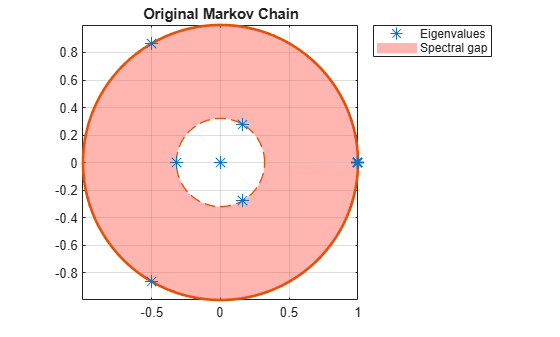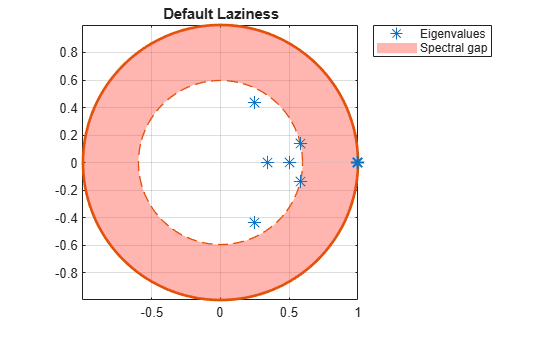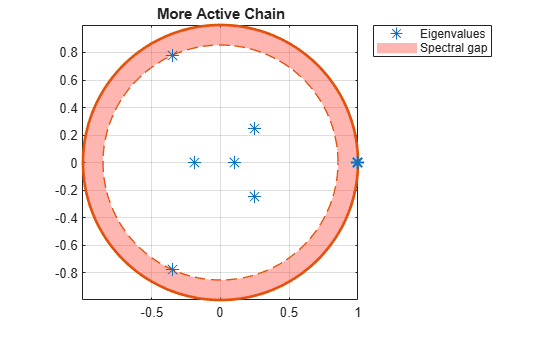# lazy

## Syntax

``lc = lazy(mc)``
``lc = lazy(mc,w)``

## Description

example

````lc = lazy(mc)` transforms the discrete-time Markov chain `mc` into the lazy chain `lc` with an adjusted state inertia.```

example

``lc = lazy(mc,w)` applies the inertial weights `w` for the transformation.`

## Examples

collapse all

Consider this three-state transition matrix.

`$P=\left[\begin{array}{ccc}0& 1& 0\\ 0& 0& 1\\ 1& 0& 0\end{array}\right].$`

Create the irreducible and periodic Markov chain that is characterized by the transition matrix P.

```P = [0 1 0; 0 0 1; 1 0 0]; mc = dtmc(P);```

At time t = 1,..., T, `mc` is forced to move to another state deterministically.

Determine the stationary distribution of the Markov chain and whether it is ergodic.

`xFix = asymptotics(mc)`
```xFix = 1×3 0.3333 0.3333 0.3333 ```
`isergodic(mc)`
```ans = logical 0 ```

`mc` is irreducible and not ergodic. As a result, `mc` has a stationary distribution, but it is not a limiting distribution for all initial distributions.

Show why `xFix` is not a limiting distribution for all initial distributions.

```x0 = [1 0 0]; x1 = x0*P```
```x1 = 1×3 0 1 0 ```
`x2 = x1*P`
```x2 = 1×3 0 0 1 ```
`x3 = x2*P`
```x3 = 1×3 1 0 0 ```
`sum(x3 == x0) == mc.NumStates`
```ans = logical 1 ```

The initial distribution is reached again after several steps, which implies that the subsequent state distributions cycle through the same sets of distributions indefinitely. Therefore, `mc` does not have a limiting distribution.

Create a lazy version of the Markov chain `mc`.

`lc = lazy(mc)`
```lc = dtmc with properties: P: [3x3 double] StateNames: ["1" "2" "3"] NumStates: 3 ```
`lc.P`
```ans = 3×3 0.5000 0.5000 0 0 0.5000 0.5000 0.5000 0 0.5000 ```

`lc` is a `dtmc` object. At time t = 1,..., T, `lc` "flips a fair coin". It remains in its current state if the "coin shows heads" and transitions to another state if the "coin shows tails".

Determine the stationary distribution of the lazy chain and whether it is ergodic.

`lcxFix = asymptotics(lc)`
```lcxFix = 1×3 0.3333 0.3333 0.3333 ```
`isergodic(lc)`
```ans = logical 1 ```

`lc` and `mc` have the same stationary distributions, but only `lc` is ergodic. Therefore, the limiting distribution of `lc` exists and is equal to its stationary distribution.

Consider this theoretical, right-stochastic transition matrix of a stochastic process.

`$P=\left[\begin{array}{ccccccc}0& 0& 1/2& 1/4& 1/4& 0& 0\\ 0& 0& 1/3& 0& 2/3& 0& 0\\ 0& 0& 0& 0& 0& 1/3& 2/3\\ 0& 0& 0& 0& 0& 1/2& 1/2\\ 0& 0& 0& 0& 0& 3/4& 1/4\\ 1/2& 1/2& 0& 0& 0& 0& 0\\ 1/4& 3/4& 0& 0& 0& 0& 0\end{array}\right].$`

Create the Markov chain that is characterized by the transition matrix P.

```P = [ 0 0 1/2 1/4 1/4 0 0 ; 0 0 1/3 0 2/3 0 0 ; 0 0 0 0 0 1/3 2/3; 0 0 0 0 0 1/2 1/2; 0 0 0 0 0 3/4 1/4; 1/2 1/2 0 0 0 0 0 ; 1/4 3/4 0 0 0 0 0 ]; mc = dtmc(P);```

Plot the eigenvalues of the transition matrix on the complex plane.

```figure; eigplot(mc); title('Original Markov Chain')```Three eigenvalues have modulus one, which indicates that the period of `mc` is three.

Create lazy versions of the Markov chain `mc` using various inertial weights. Plot the eigenvalues of the lazy chains on separate complex planes.

```w2 = 0.1; % More active Markov chain w3 = 0.9; % Lazier Markov chain w4 = [0.9 0.1 0.25 0.5 0.25 0.001 0.999]; % Laziness differs between states lc1 = lazy(mc); lc2 = lazy(mc,w2); lc3 = lazy(mc,w3); lc4 = lazy(mc,w4); figure; eigplot(lc1); title('Default Laziness');``````figure; eigplot(lc2); title('More Active Chain');``````figure; eigplot(lc3); title('Lazier Chain');``````figure; eigplot(lc4); title('Differing Laziness Levels');```All lazy chains have only one eigenvalue with modulus one. Therefore, they are aperiodic. The spectral gap (distance between inner and outer circle) determines the mixing time. Observe that all lazy chains take longer to mix than the original Markov chain. Chains with different inertial weights than the default take longer to mix than the default lazy chain.

## Input Arguments

collapse all

Discrete-time Markov chain with `NumStates` states and transition matrix `P`, specified as a `dtmc` object. `P` must be fully specified (no `NaN` entries).

Inertial weights, specified as a numeric scalar or vector of length `NumStates`. Values must be between `0` and `1`.

• If `w` is a scalar, `lazy` applies it to all states. That is, the transition matrix of the lazy chain (`lc.P`) is the result of the linear transformation

`${P}_{\text{lazy}}=\left(1-w\right)P+wI.$`

P is `mc.P` and I is the `NumStates`-by-`NumStates` identity matrix.

• If `w` is a vector, `lazy` applies the weights state by state (row by row).

Data Types: `double`

## Output Arguments

collapse all

Discrete-time Markov chain, returned as a `dtmc` object. `lc` is the lazy version of `mc`.

collapse all

### Lazy Chain

A lazy version of a Markov chain has, for each state, a probability of staying in the same state equal to at least 0.5.

In a directed graph of a Markov chain, the default lazy transformation ensures self-loops on all states, eliminating periodicity. If the Markov chain is irreducible, then its lazy version is ergodic. See `graphplot`.

 Gallager, R.G. Stochastic Processes: Theory for Applications. Cambridge, UK: Cambridge University Press, 2013.